# 滑动窗口算法（Sliding Window Algorithm）

Sliding window algorithm is used to perform required operation on specific window size of given large buffer or array.

This technique shows how a nested for loop in few problems can be converted to single for loop and hence reducing the time complexity.

# 基本示例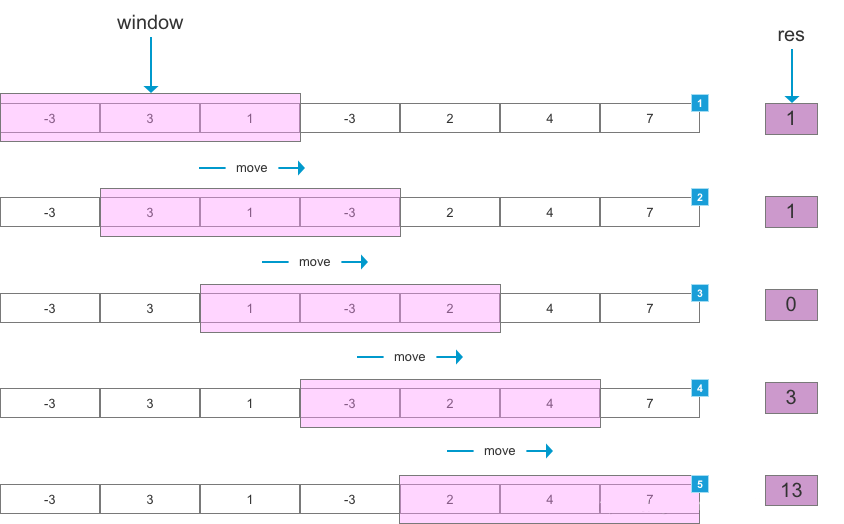# 什么是滑动窗口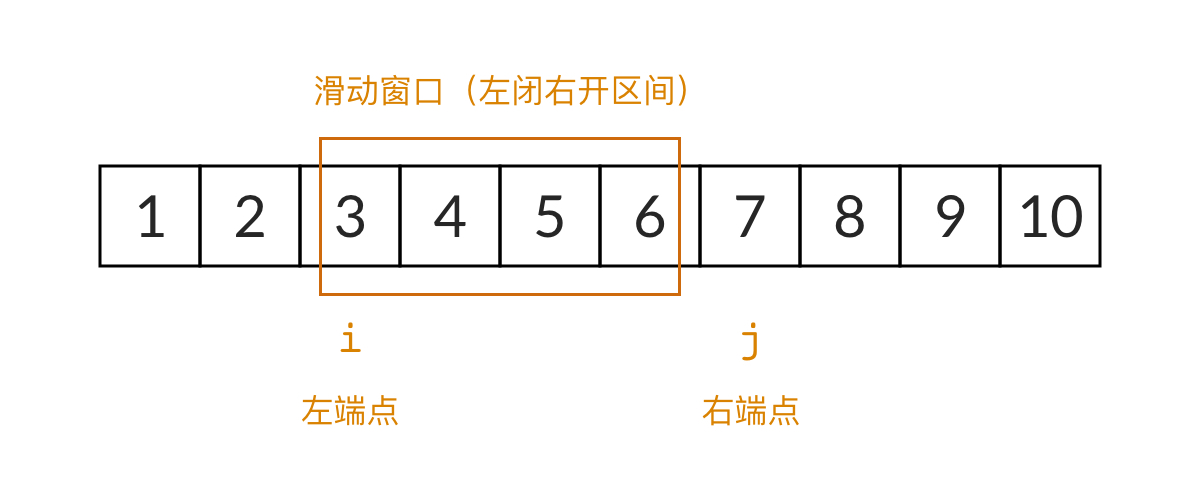# 如何用滑动窗口解这道题

• 第一个问题，窗口何时扩大，何时缩小？
• 第二个问题，滑动窗口能找到全部的解吗？

• 当窗口的和小于 target 的时候，窗口的和需要增加，所以要扩大窗口，窗口的右边界向右移动
• 当窗口的和大于 target 的时候，窗口的和需要减少，所以要缩小窗口，窗口的左边界向右移动
• 当窗口的和恰好等于 target 的时候，我们需要记录此时的结果。设此时的窗口为 $$[i, j)$$，那么我们已经找到了一个 i 开头的序列，也是唯一一个 i 开头的序列，接下来需要找 i+1 开头的序列，所以窗口的左边界要向右移动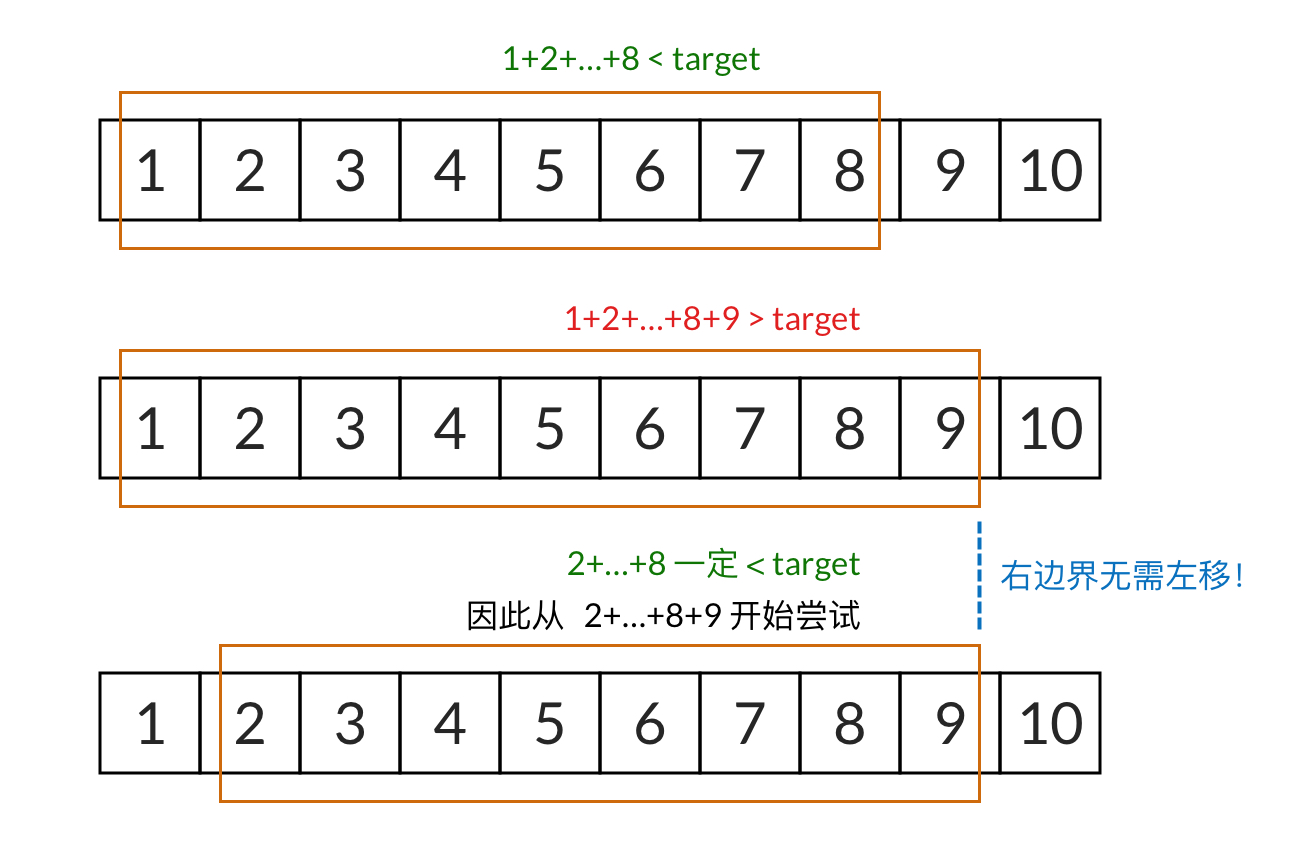# 滑动窗口三步走

• 前闭后开，left 元素包含在滑动窗口内，right 元素不包含在滑动窗口内
• 使用 right 来进行遍历元素，满足条件就 right++，加入滑动窗口
• 滑动窗口 left、right 只能前进，不能后退，所以一般只用比较最大值即可

• 右边界向右移动（扩大窗口）：（双指针）right++; 或者 （双端队列）linkedList.addFirst(right);
• 左边界向右移动（缩小窗口）：（双指针）left++; 或者 （双端队列）linkedList.removeLast();

## 1. 明确循环条件

1.明确循环条件：right 如何遍历数组？什么时候保持循环，什么时候结束循环？

## 2. 寻找扩缩条件

2.寻找扩缩条件：窗口何时扩大，何时缩小？

## 3. 完成目标功能

3.完成目标功能：当滑动窗口满足条件时，完成目标功能。

# 滑动窗口法的大体框架

• 滑动：说明这个窗口是移动的，也就是移动是按照一定方向来的。

• 窗口：窗口大小并不是固定的，可以不断扩容直到满足一定的条件；也可以不断缩小，直到找到一个满足条件的最小窗口；当然也可以是固定大小。

1. 我们在字符串 S 中使用双指针中的左右指针技巧，初始化 left = right = 0，把索引闭区间 [left, right] 称为一个“窗口”。

2. 我们先不断地增加 right 指针扩大窗口 [left, right]，直到窗口中的字符串符合要求（包含了 T 中的所有字符）。

3. 此时，我们停止增加 right，转而不断增加 left 指针缩小窗口 [left, right]，直到窗口中的字符串不再符合要求（不包含 T 中的所有字符了）。同时，每次增加 left，我们都要更新一轮结果。

4. 重复第 2 和第 3 步，直到 right 到达字符串 S 的尽头。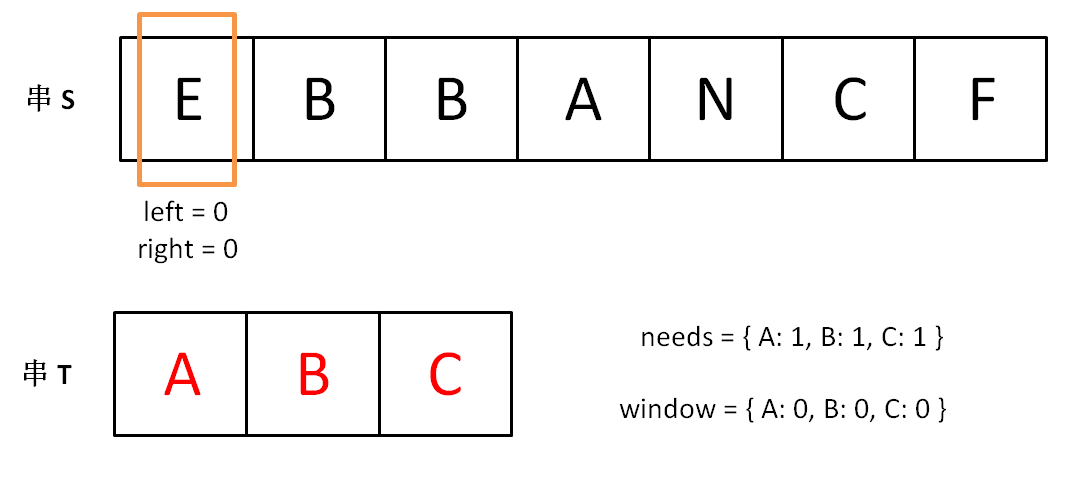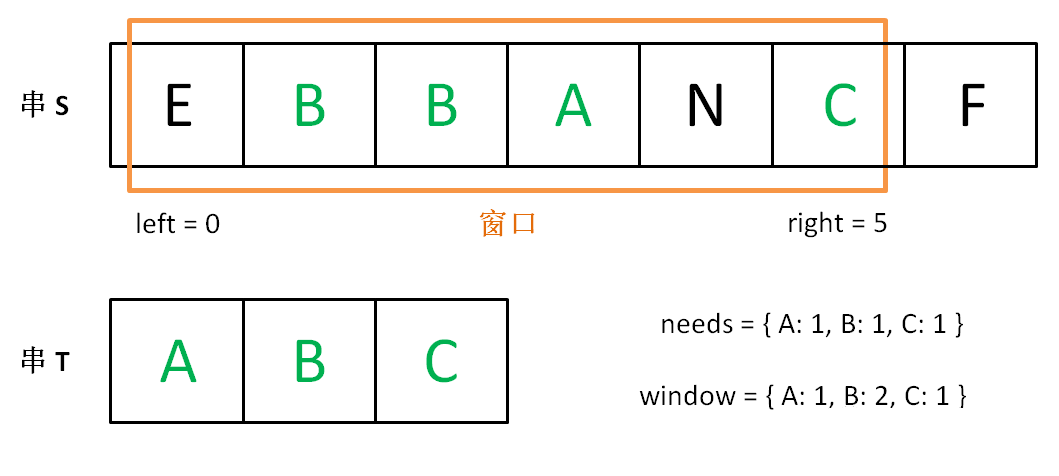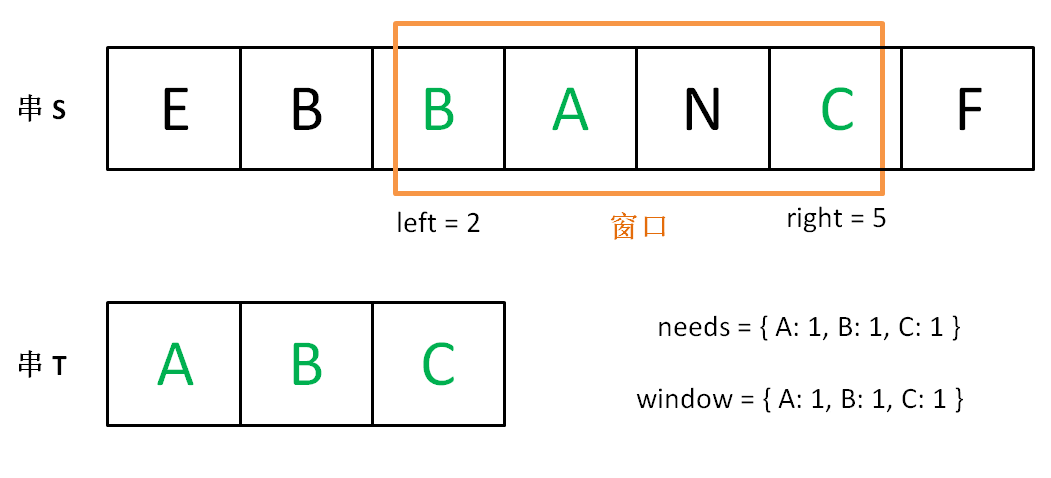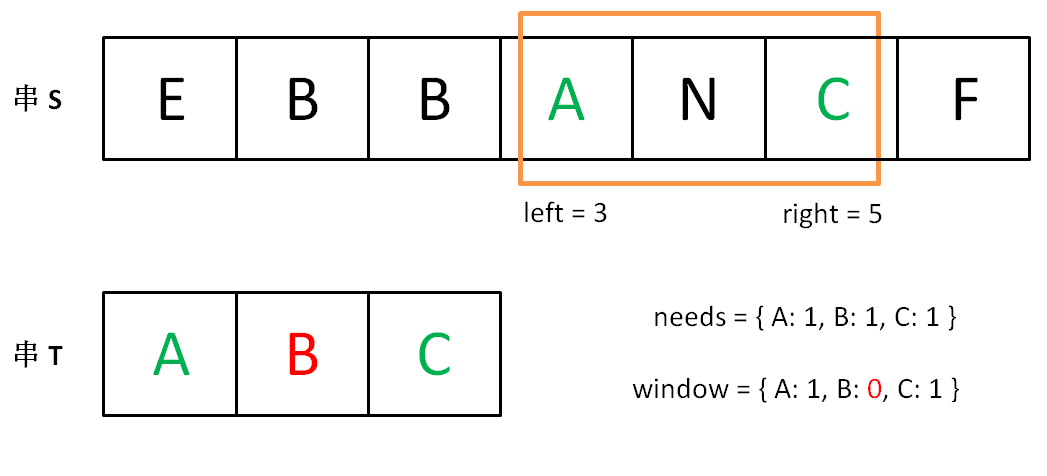int slidingWindow() {
string s, t;
// 在 s 中寻找 t 的“最小覆盖子串”
int left = 0, right = 0;
string res = s;

while(right < s.size()) {
right++;
// 如果符合要求，说明窗口构造完成，移动 left 缩小窗口
while (window 符合要求) {
// 如果这个窗口的子串更短，则更新 res
res = minLen(res, window);
window.remove(s[left]);
left++;
}
}
return res;
}

int slidingWindow() {
// 固定窗口大小为 k
string s;
// 在 s 中寻找窗口大小为 k 时的所包含最大元音字母个数
int  right = 0;
while(right < s.size()) {
right++;
// 如果符合要求，说明窗口构造完成，
if (right>=k) {
// 这是已经是一个窗口了，根据条件做一些事情
// ... 可以计算窗口最大值等
// 最后不要忘记把 right -k 位置元素从窗口里面移除
}
}
return res;
}

# 实例1

## 1208. 尽可能使字符串相等

class Solution {
public int equalSubstring(String s, String t, int maxCost) {
int left = 0, right =0;
int sum = 0;
int res = 0;
// 构造窗口
while (right < s.length()) {
sum += Math.abs(s.charAt(right) - t.charAt(right));
right++;
// 窗口构造完成，这时候要根据条件当前的窗口调整窗口大小
while (sum > maxCost) {
sum -=  Math.abs(s.charAt(left) - t.charAt(left));
left++;
}
// 记录此时窗口的大小
res = Math.max(res, right -left);
}
return res;
}
}

## 239. 滑动窗口最大值

————— —–
[1 3 -1] -3 5 3 6 7 3
1 [3 -1 -3] 5 3 6 7 3
1 3 [-1 -3 5] 3 6 7 5
1 3 -1 [-3 5 3] 6 7 5
1 3 -1 -3 [5 3 6] 7 6
1 3 -1 -3 5 [3 6 7] 7

1 <= nums.length <= 10^5
-10^4 <= nums[i] <= 10^4
1 <= k <= nums.length

class Solution {
public static int[] maxSlidingWindow(int[] nums, int k) {
int right =0;
int[] res = new int[nums.length -k +1];
int index=0;
// 开始构造窗口
while (right < nums.length) {
// 这里的list的首位必须是窗口中最大的那位
while (!list.isEmpty() && nums[right] > list.peekLast()) {
list.removeLast();
}
// 不断添加
right++;
// 构造窗口完成，这时候需要根据条件做一些操作
if (right >= k){
res[index++]=list.peekFirst();
// 如果发现第一个已经在窗口外面了，就移除
if(list.peekFirst() == nums[right-k]) {
list.removeFirst();
}
}
}
return res;
}
} 

## 1456. 定长子串中元音的最大数目

1 <= s.length <= 10^5
s 由小写英文字母组成
1 <= k <= s.length

## 解答

class Solution {
public int maxVowels(String s, int k) {
int right =0;
int sum = 0;
int max = 0;
while (right < s.length()) {
sum += isYuan(s.charAt(right)) ;
right++;
if (right >=k) {
max = Math.max(max, sum);
sum -= isYuan(s.charAt(right-k));
}
}
return max;
}

public int isYuan(char s) {
return s=='a' || s=='e' ||s=='i' ||s=='o' ||s=='u' ? 1:0;
}
}

# 实例2

## 剑指Offer57.和为s的连续正数序列（简单）

### 答案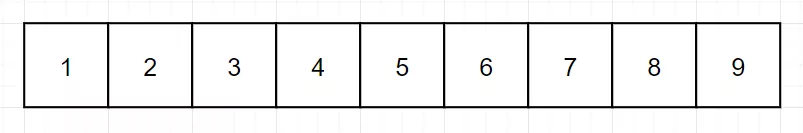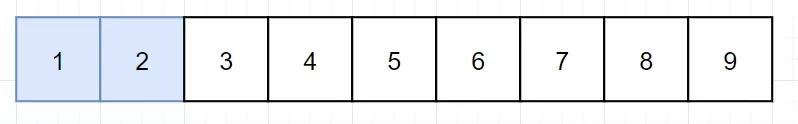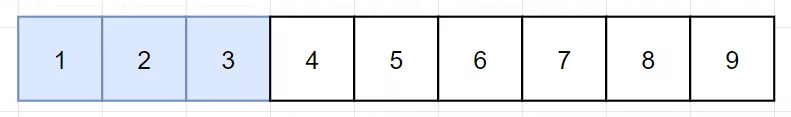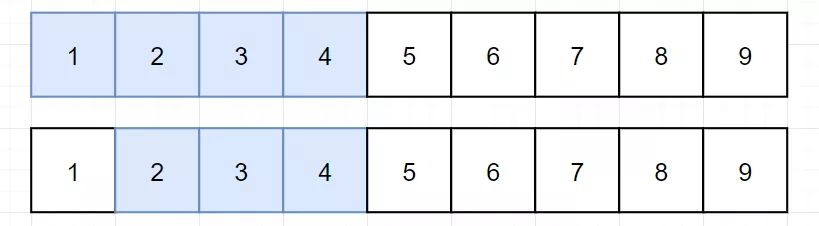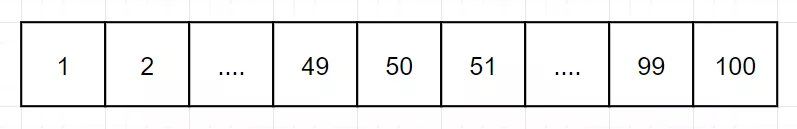//java
class Solution {
public int[][] findContinuousSequence(int target) {
List<int[]> res = new ArrayList<>();
int i = 1;
int j = 1;
int win = 0;
while (i <= target / 2) {
if (win < target) {
win += j;
j++;
} else if (win > target) {
win -= i;
i++;
} else {
int[] arr = new int[j-i];
for (int k = i; k < j; k++) {
arr[k-i] = k;
}
win -= i;
i++;
}
}
return res.toArray(new int[res.size()][]);
}
}

### 答案2

#### 什么是滑动窗口#### 如何用滑动窗口解这道题

• 第一个问题，窗口何时扩大，何时缩小？
• 第二个问题，滑动窗口能找到全部的解吗？

• 当窗口的和小于 target 的时候，窗口的和需要增加，所以要扩大窗口，窗口的右边界向右移动
• 当窗口的和大于 target 的时候，窗口的和需要减少，所以要缩小窗口，窗口的左边界向右移动
• 当窗口的和恰好等于 target 的时候，我们需要记录此时的结果。设此时的窗口为 $$[i, j)$$，那么我们已经找到了一个 i 开头的序列，也是唯一一个 i 开头的序列，接下来需要找 i+1 开头的序列，所以窗口的左边界要向右移动class Solution {
public int[][] findContinuousSequence(int target) {

// 这得从1开始看起
// 如果 滑动窗口的数字和sum == target 那么就可以加入列表，算一个
// 如果大于，那就前进 left，
// 如果小于，前进 right

int left = 1;
int right = 1;
int sum = 0;

List<int[]> list = new ArrayList<>();

// while (right <= target) {
while (left <= target / 2) {

if (sum == target) {

int[] arr = new int[right - left];
for (int i = left; i < right; i++) {
arr[i - left] = i;
}

// 通过了就左边界前进，继续遍历
sum -= left;
left++;
} else if (sum < target) {
sum += right;
right++;
} else if (sum > target) {
sum -= left;
left++;
}
}

// return list.toArray();   // 这样是不行的，不然返回的是Object[]数组，得指明数组类型
return list.toArray(new int[list.size()][]);
}
}

## 3. 无重复字符的最长子串

### 答案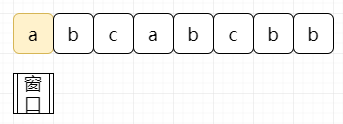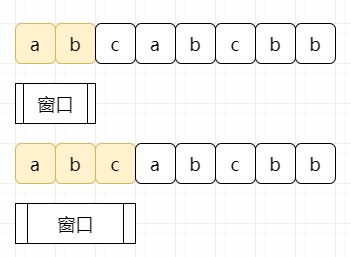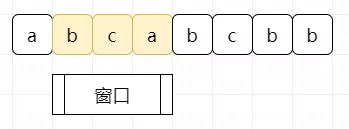//java
public class Solution {
public static int lengthOfLongestSubstring(String s) {
int n = s.length();
Set<Character> set = new HashSet<>();
int result = 0, left = 0, right = 0;
while (left < n && right < n) {
//charAt：返回指定位置处的字符
if (!set.contains(s.charAt(right))) {
right++;
result = Math.max(result, right - left);
} else {
set.remove(s.charAt(left));
left++;
}
}
return result;
}
}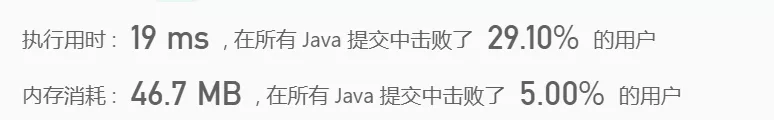class Solution {
public int lengthOfLongestSubstring(String s) {

int left = 0;
int right = 0;  // 使用右边界来遍历数组
int result = 0; // 用来存放最长子串的长度

char c = 0; // 用来遍历字符串中每个字符

Map<Character, Integer> map = new HashMap<>();

// 前闭后开，滑动窗口
// 每次遍历都添加频度
// 如果不重复就加入哈希表，右边界前进，比较最大长度
// 如果重复，删除哈希表，左边界前进

while (right < s.length()) {
// 使用右边界来遍历数组，后面再判断是否加入哈希表中
c = s.charAt(right);
int count = map.getOrDefault(c, 0) + 1;

if (count > 1) {    // 重复，左边界前进，哈希表删除
map.put(s.charAt(left), map.get(s.charAt(left)) - 1);
left++;
} else {    // 右边界前进，哈希表增加
map.put(c, count);
right++;
result = result > (right - left)? result : (right - left);
}

}
return result;
}
}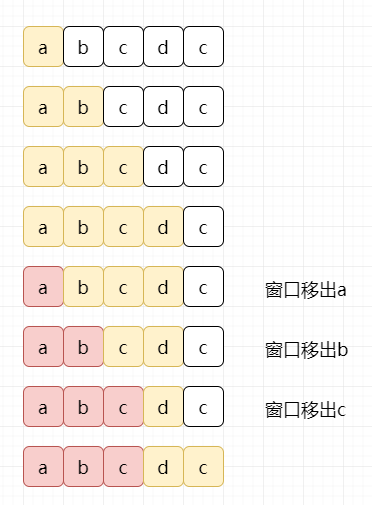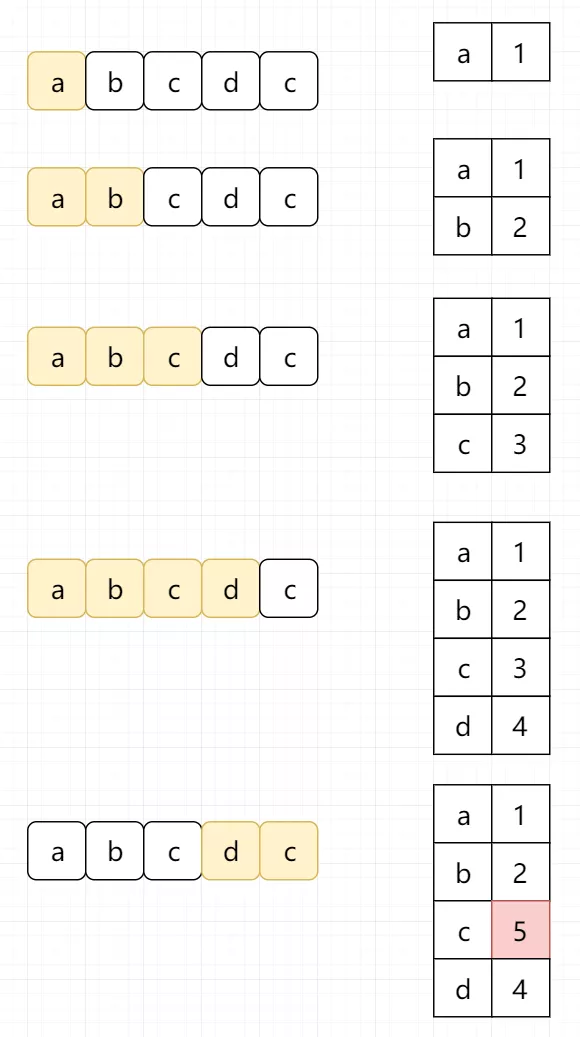//java
public class Solution {
public static int lengthOfLongestSubstring(String s) {
int n = s.length(), result = 0;
Map<Character, Integer> map = new HashMap<>();
for (int right = 0, left = 0; right < n; right++) {
if (map.containsKey(s.charAt(right))) {
left = Math.max(map.get(s.charAt(right)), left);
}
result = Math.max(result, right - left + 1);
map.put(s.charAt(right), right + 1);
}
return result;
}
}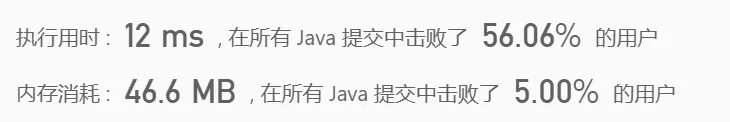//java
// 上面的哈希表记录的是（字符，频数）
// 这里的哈希表记录的是（字符，出现索引+1）
public class Solution {
public static int lengthOfLongestSubstring(String s) {
int n = s.length(), result = 0;
Map<Character, Integer> map = new HashMap<>();

int left = 0;
int right = 0;  // 使用右边界来遍历数组

char c = 0;

while (right < s.length()) {

c = s.charAt(right);
int count = map.getOrDefault(c, -1);    // -1代表没有出现过

if (count == -1) {  // 没有出现过
map.put(c, right + 1);
right++;
result = Math.max(result, right - left);
} else {    // 出现过
left = Math.max(count, left);   // 这里需要着重注意，因为滑动窗口left只能前进，不能倒退回去，只能取最大的
map.put(c, right + 1);
right++;
result = Math.max(result, right - left);
}
}

// for (int right = 0, left = 0; right < n; right++) {
//     if (map.containsKey(s.charAt(right))) {
//         left = Math.max(map.get(s.charAt(right)), left);
//     }
//     result = Math.max(result, right - left + 1);
//     map.put(s.charAt(right), right + 1);
// }
return result;
}
}

//java
class Solution {
public int lengthOfLongestSubstring(String s) {
int len = s.length();
int result = 0;
int[] charIndex = new int;
for (int left = 0, right = 0; right < len; right++) {
char c = s.charAt(right);
left = Math.max(charIndex[c], left);
result = Math.max(result, right - left + 1);
charIndex[c] = right + 1;
}
return result;
}
}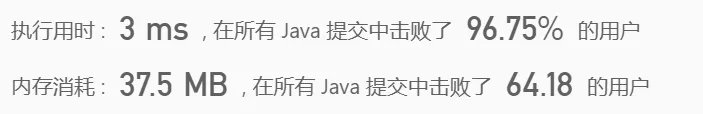## 438. 找到字符串中所有字母异位词

s: “cbaebabacd” p: “abc”

[0, 6]

s: “abab” p: “ab”

[0, 1, 2]

### 答案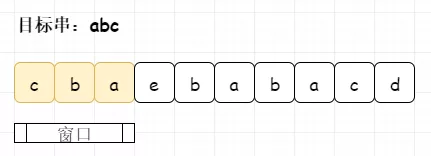pArr为目标串数组，sArr为窗口数组。我们发现初始化数组，本身就满足，记录下来。（这里图示用map模拟数组，便于理解）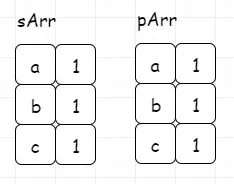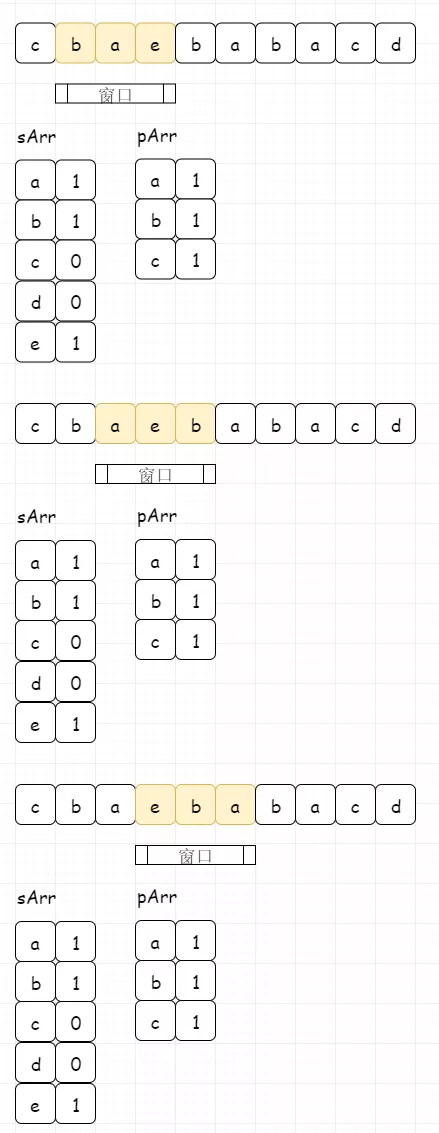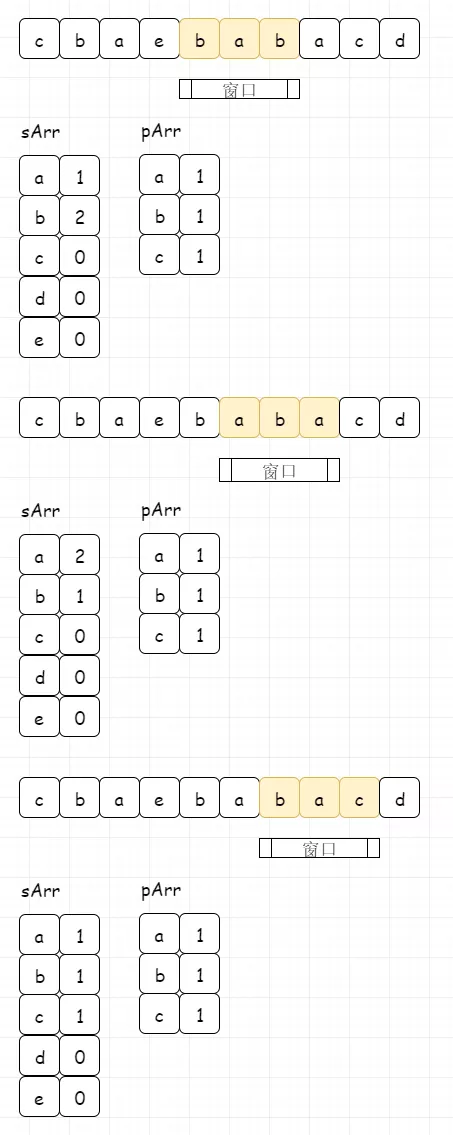class Solution {

public List<Integer> findAnagrams(String s, String p) {

if (s == null || p == null || s.length() < p.length()) return new ArrayList<>();

List<Integer> list = new ArrayList<>();

int[] pArr = new int;
int pSize = p.length();
int[] sArr = new int;

for (int i = 0; i < p.length(); i++) {
sArr[s.charAt(i) - 'a']++;
pArr[p.charAt(i) - 'a']++;
}

for (int i = 0; i < p.length(); i++) {
int index = p.charAt(i) - 'a';
if (pArr[index] == sArr[index])
pSize--;
}

int i = 0;
int j = p.length();

// 窗口大小固定为p的长度
while (j < s.length()) {
if (isSame(pArr, sArr))
//sArr[s.charAt(i) - 'a']-- 左指针位置处字母减1
sArr[s.charAt(i) - 'a']--;
i++;
//sArr[s.charAt(j) - 'a']++ 右指针位置处字母加1
sArr[s.charAt(j) - 'a']++;
j++;
}

if (isSame( pArr, sArr))

return list;
}

public boolean isSame(int[] arr1, int[] arr2) {
for (int i = 0; i < arr1.length; ++i)
if (arr1[i] != arr2[i])
return false;
return true;
}
}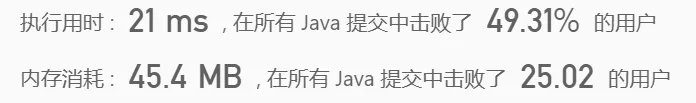class Solution {
public List<Integer> findAnagrams(String s, String p) {

// 固定窗口大小为p.length() - 1
int left = 0;
int right = p.length();

List<Integer> list = new ArrayList<>();

Map<Character, Integer> mapP = new HashMap<>();
Map<Character, Integer> mapS = new HashMap<>();

// 将p的所有字符放入哈希表
for (int i = 0; i < p.length(); i++) {

char c = p.charAt(i);
int count = mapP.getOrDefault(c, 0) + 1;

mapP.put(c, count);
}

while (right <= s.length()) {

// 从left到right，遍历窗口
for (int i = left; i < right; i++) {

char c = s.charAt(i);
int count = mapS.getOrDefault(c, 0) + 1;

mapS.put(c, count);
}

if (mapP.equals(mapS)) {
}

// 无论如何都要前进
left++;
right++;

// 清理一下mapS，便于下个窗口存入
mapS.clear();
}
return list;
}
}

// 上面的算法面对超长超大的字符串会超时，所以我们把哈希表换成了自己写的
class Solution {
public List<Integer> findAnagrams(String s, String p) {

int left = 0;
int right = p.length();

int[] mapP = new int;   // p的哈希表
int[] mapS = new int;   // s的哈希表

List<Integer> list = new ArrayList<>();

// 将p的所有字符放入哈希表
for (int i = 0; i < p.length(); i++) {
mapP[p.charAt(i) - 'a']++;
}

// 由于前闭后开，所以right得等于s.length()才算遍历完了所有
while (right <= s.length()) {

mapS = new int;

for (int i = left; i < right; i++) {
mapS[s.charAt(i) - 'a']++;
}

if (isSame(mapP, mapS)) {
}

left++;
right++;
}
return list;
}

public boolean isSame(int[] mapP, int[] mapS) {
for (int i = 0; i < mapP.length; i++) {
if (mapP[i] != mapS[i]) {
return false;
}
}
return true;
}
}

## 239. 滑动窗口最大值（困难可不做）

[1 3 -1] -3 5 3 6 7 3

1 [3 -1 -3] 5 3 6 7 3

1 3 [-1 -3 5] 3 6 7 5

1 3 -1 [-3 5 3] 6 7 5

1 3 -1 -3 [5 3 6] 7 6

1 3 -1 -3 5 [3 6 7] 7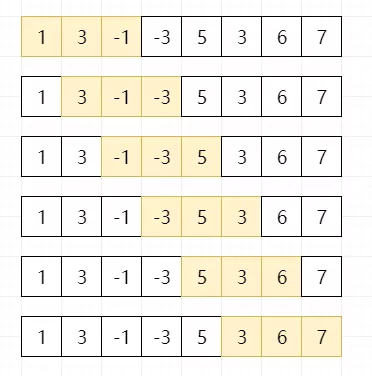//java
class Solution {
public int[] maxSlidingWindow(int[] nums, int k) {
int len = nums.length;
if (len * k == 0) return new int;
int [] win = new int[len - k + 1];
//遍历所有的滑动窗口
for (int i = 0; i < len - k + 1; i++) {
int max = Integer.MIN_VALUE;
//找到每一个滑动窗口的最大值
for(int j = i; j < i + k; j++) {
max = Math.max(max, nums[j]);
}
win[i] = max;
}
return win;
}
}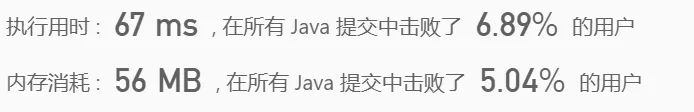It’s Bullshit！结果令我们很不满意，时间复杂度达到了O(LK)，如果面试问到这道题，基本上只写出这样的代码，一定就挂掉了。那我们怎么样优化时间复杂度呢？有没有可以O(L)的实现呢？=_=

### 线性题解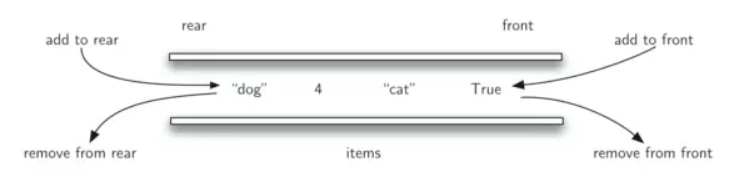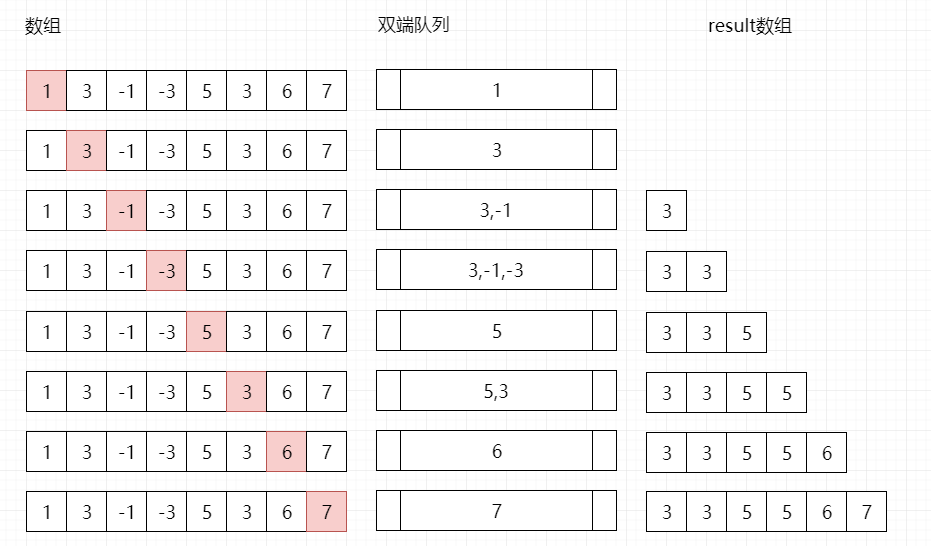（个人认为我画的这个图是目前全网对于双端队列本题解法比较清晰的一个…所以我觉得如果不点个赞的话…晤..）

//go
func maxSlidingWindow(nums []int, k int) []int {
if len(nums) == 0 {
return []int{}
}
//用切片模拟一个双端队列
queue := []int{}
result := []int{}
for i := range nums {
for i > 0 && (len(queue) > 0) && nums[i] > queue[len(queue)-1] {
//将比当前元素小的元素祭天
queue = queue[:len(queue)-1]
}
//将当前元素放入queue中
queue = append(queue, nums[i])
if i >= k && nums[i-k] == queue {
//维护队列，保证其头元素为当前窗口最大值
queue = queue[1:]
}
if i >= k-1 {
//放入结果数组
result = append(result, queue)
}
}
return result
}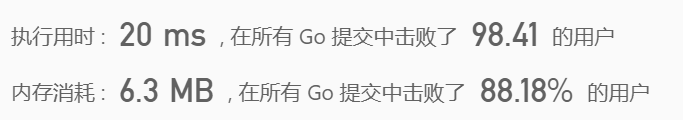Perfact~题目完成！看着一下子超越百分之99的用户，是不是感觉很爽呢~

//Java
class Solution {
public int[] maxSlidingWindow(int[] nums, int k) {
if(nums == null || nums.length < 2) return nums;
// 双向队列 保存当前窗口最大值的数组位置 保证队列中数组位置的数值按从大到小排序
// 结果数组
int[] result = new int[nums.length-k+1];
// 遍历nums数组
for(int i = 0;i < nums.length;i++){
// 保证从大到小 如果前面数小则需要依次弹出，直至满足要求
while(!queue.isEmpty() && nums[queue.peekLast()] <= nums[i]){
queue.pollLast();
}
// 添加当前值对应的数组下标
}